## Is it a correct result that the limit of log(x)/x ...

The following expressionreturns undefined in Maple 2022.

I am not certain about if this limit exists, but shouldn't it be -infinity?

## Famous Constants on Maple...

For famous constants like pi and e, are the values stored or are they calculated?

## How can I solve this units problem?...(1)(2)(3)(4)(5)Warning, units problem, not enough information to unambiguously deduce the units of the variables {t}; proceeding as if dimensionless

How can I provide the information to Maple that the unit of t is s?

Update: Temporarily disabling the warning would also be an option for the above case.

## calculation of Major Axis and Minor Axis of an ell...

How to improve this program ? Thank you.

restart;
Equation de la conique
eqcon := (45 - 27*cos(alpha))*x^2 - 54*sin(alpha)*x*y + (45 + 27*cos(alpha))*y^2 - 8;
Delta := (-54*sin(alpha))^2 - 4*(45 - 27*cos(alpha))*(45 + 27*cos(alpha));
expand(%);
simplify(%);
Discriminant : Δ<0 ce qui correxpond à une ellipse
Eq := simplify(expand(subs(x = cos(alpha/2)*X - sin(alpha/2)*Y, y = sin(alpha/2)*X + cos(alpha/2)*Y, eqcon)));
kx := coeff(Eq, X, 2);
ky := coeff(Eq, Y, 2);
k := -tcoeff(Eq);

EQ := X^2/(sqrt(1/kx^2)*k) + Y^2/(sqrt(1/ky^2)*k) = 1;
Calcul du grand et du petit axe
a := 1/sqrt(coeff(lhs(EQ), X, 2));
b := 1/sqrt(coeff(lhs(EQ), Y, 2));
print(X^2/('a^2') + Y^2/('b^2') = 1);

## How to compute the restricted-edge-connectivity of...

！Edits: I have found  a existing polynomial algorithm, but I still have difficulty implementing it. 2022/10/26

The edge connectivity of a connected graph G  is the minimum number of edges whose deletion from the graph G disconnects G. Below we are concerned with a particular kind of edge-cut.

• For a connected graph G=(V ,E), an edge set S ⊂ E is a restricted-edge-cut, if G−S is disconnected and every connected component of  G−S has at least 2 vertices.

Clearly, a restricted-edge-cut is an edge cut with a special requirement.

• The restricted-edge-connectivity of G, denoted by κres(G), is defined as the cardinality of a minimum restricted-edge-cut.

For example, a graph g is as follows.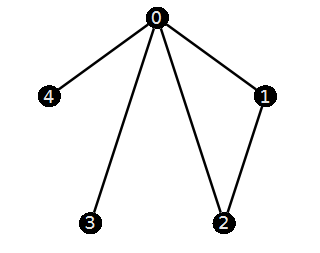Clearly, its edge-connectivity is 1 since (0,3) or (0,4) is a edge cut of g. But we can find that  if we remove the edge (0,3), then "3" is a isolated vertex. Similarly, "4" is a isolated vertex if we remove  (0,4). It is not difficult to find g has exactly the two cut-edges (0,3) and (0,4).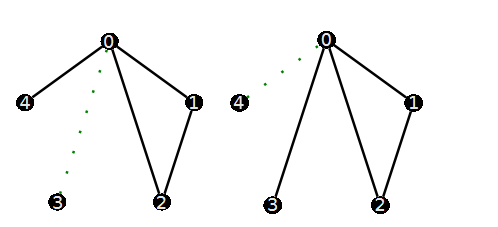Based on the definition of the restricted-edge-cuts, neither {(0,3)} nor  {(0,4)} are restricted-edge-cuts A minimum restricted-edge-cut is {(0,1),(0,2)} since every connected component of G-{(0,1),(0,2)} has  (at least) 2 vertices.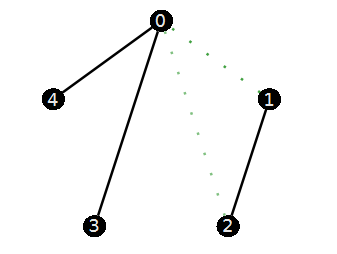So  κres(g) is 2.  My problem is:

Given a graph G, how to calculate the restricted-edge-connectivity of  G?  Moreover, how to find a minimum restricted-edge-cut?

A specific graph that I want to test is as follows. (it has 16 vertices and 56 edges.) I would like to calculate its restricted-edge-connectivity and find a minimum restricted-edge-cut.

```g:=ConvertGraph("O~tIID@wL~j`PbOqgLJ@p");
vertexcolor="Black",edgethickness=2])
```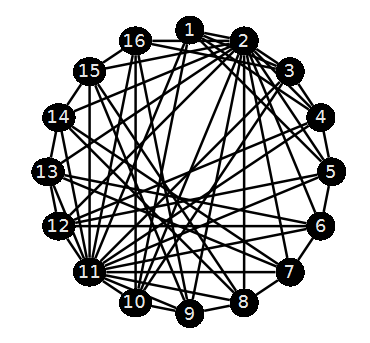`EdgeConnectivity(g) `

6

One option I came up with is to find all 6-edge subsets first. Test if they satisfy  the restricted condition (one by one). Then continue to increase to 7 or more. But this violent calculation may get stuck in the first step. That is, test all the minimum edge cut sets (Note that we will consider 32468436 edge-subsets!) I was referring to the efficiency aspect.

```with(Iterator):
C:=Combination(58,6):
K:=Edges(g):
#sub:=seq( K[[seq(c[]+1)]], c=C); # do not run this code since it has 32468436 members.```

Any language is acceptable.( C or C++, Python. )

PS: Some time ago, I also asked a related question (but with some differences) on mathematica stack （Find all the minimum edge cuts of a graph）. Although Bob Hanlon  gave a reply, the actual result is not good.

Edits: The following literature gives a polynomial algorithm for computing the restricted-edge-connectivity of a given  graph. The heart of it is to computing the least cardinality of some  edge-pairs's edge separator. I'm stuck here.

• Esfahanian A H, Hakimi S L. On computing a conditional edge-connectivity of a graph[J]. Information processing letters, 1988, 27(4): 195-199.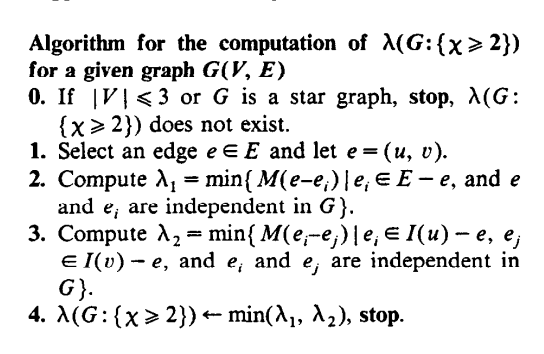How to implement this algorithm is my current concern.

## Set reduction in an array...

Hi All,

I solved a high degree polynomial equation for two variables and obtained a list of solutions as under:

{s = 0.01291429045, t = 0.06099359500}, {s = -0.6944124361, t = 0.8895072341}, {s = 0.3000821715, t = -0.06855860577}, {s = 0.1246366197, t = -0.06899397557}, {s = 43.29465387, t = -10.98020355}, {s = 7.400522990*10^6, t = -1.879497900*10^6}, {s = 7.442623977*10^6, t = -1.890190214*10^6}

In the second stage, I have to plug these solutions into another expression andsolve for second stage decision variables. However, I am only interested in the solutions where both s and t are non negative. How can I reduce the set to have a smaller subset?

Thanks for help

## referencing module members within the module...

I use to write modules with option package and store them in a .mla files.
Let's assume M is the name of the module and P1, P2, ..., PN are the procedures M contains.
Each time one of these procedures hass to call another one, I write

```Pm := proc(...)
...
a := M:-Pn(...)
...
end proc:```

```Pm := proc(...)
...
a := Pn(...)
...
end proc:```

It seemed to me that the first method sometimes saved me a lot of trouble

Is it a common/necessary/safer/useless practice to write a:=M:Pn(...) instead of a:=Pn(...) ?

## Multi-variable ...

{[[b/(sin(x-y-z))=a/(sin(z)),],[(a^(2)+b^(2)+2 abcos(x-y))cos(m(y+z)+n)=acos(mx+n)+bcos(my+n),]];

Why cannot this group of equations be solved in Maple software?

## Procedures using other saved procedures...

I have 5 procedures saved in my library. Say Proc1, Proc2, Proc3, Proc4 and Proc5. They all work ok.

Proc5 calls Proc4 which calls Proc3 which calls Proc2 which calls Proc1. That is fine if I read them all in to the dodument.

Is it ok ok to put read commands in procedures 5..2 so I don't have to manually load them all myself into a new document or is there a better way to do this. These procedures are not part of a package.
I hace set

```currentdir();
"C:\Users\Ronan\Documents\maple\Alibrary\Procedures"

```

which is where the procedures are stored. If I just load Proc5 it doesn't find the other procedures

## solve equations issue (15equations with 16 varible...

dear all,

I am trying to use the solve to get symbolic answer.

to be simple, here is issue:

I have 15equations with 16 varibles.

the 15 equation as follows:

first 4 equation set

```equList_Ya :=

[ Va1 Ya11 + Va2 Ya12 + Va3 Ya13 + Va4 Ya14 = Ia1,

Va1 Ya21 + Va2 Ya22 + Va3 Ya23 + Va4 Ya24 = Ia2,

Va1 Ya31 + Va2 Ya32 + Va3 Ya33 + Va4 Ya34 = Ia3,

Va1 Ya41 + Va2 Ya42 + Va3 Ya43 + Va4 Ya44 = Ia4]```

2nd 4 equation set

```equList_Yb :=

[ Vb1 Yb11 + Vb2 Yb12 + Vb3 Yb13 + Vb4 Yb14 = Ib1,

Vb1 Yb21 + Vb2 Yb22 + Vb3 Yb23 + Vb4 Yb24 = Ib2,

Vb1 Yb31 + Vb2 Yb32 + Vb3 Yb33 + Vb4 Yb34 = Ib3,

Vb1 Yb41 + Vb2 Yb42 + Vb3 Yb43 + Vb4 Yb44 = Ib4]```

the 3rd 4 equation set

```equList_ConnectCondition :=

[Ia3 = -Ib1, Ia4 = -Ib2, Va3 = Vb1,  Va4 = Vb2]```

the 4th 3 equation set

` equList_Y11Condition := [Va2 = 0, Vb3 = 0, Vb4 = 0]`

so total equation number is 4+4+4+3=15

```equList_Y11all := {Ia3 = -Ib1, Ia4 = -Ib2, Va2 = 0, Va3 = Vb1, Va4 = Vb2, Vb3 = 0,
Vb4 = 0, Va1*Ya11 + Va2*Ya12 + Va3*Ya13 + Va4*Ya14 = Ia1,
Va1*Ya21 + Va2*Ya22 + Va3*Ya23 + Va4*Ya24 = Ia2,
Va1*Ya31 + Va2*Ya32 + Va3*Ya33 + Va4*Ya34 = Ia3,
Va1*Ya41 + Va2*Ya42 + Va3*Ya43 + Va4*Ya44 = Ia4,
Vb1*Yb11 + Vb2*Yb12 + Vb3*Yb13 + Vb4*Yb14 = Ib1,
Vb1*Yb21 + Vb2*Yb22 + Vb3*Yb23 + Vb4*Yb24 = Ib2,
Vb1*Yb31 + Vb2*Yb32 + Vb3*Yb33 + Vb4*Yb34 = Ib3,
Vb1*Yb41 + Vb2*Yb42 + Vb3*Yb43 + Vb4*Yb44 = Ib4}```

the 16 varible is

```var_Y11 :=

[ Va1, Va2, Va3, Va4,

Ia1, Ia2, Ia3, Ia4,

Vb1, Vb2, Vb3, Vb4,

Ib1, Ib2, Ib3, Ib4]```

I hope to get Ia1=f(Va1) to calculate Y11(=Ia1/Va1)

but the solve give nothing:

`Y11_all := solve(equList_Y11all, {Ia1}, symbolic = true);                        `

Y11_all := NULL

Question1: why the above solve output nothing???

since not work,so I try another way:

```polys_Y11 := map(lhs - rhs = 0, equList_Y11all);
polys_Y11 := {Va2 = 0, Vb3 = 0, Vb4 = 0, Ia3 + Ib1 = 0,

Ia4 + Ib2 = 0, Va3 - Vb1 = 0, Va4 - Vb2 = 0,

Va1 Ya11 + Va2 Ya12 + Va3 Ya13 + Va4 Ya14 - Ia1 = 0,

Va1 Ya21 + Va2 Ya22 + Va3 Ya23 + Va4 Ya24 - Ia2 = 0,

Va1 Ya31 + Va2 Ya32 + Va3 Ya33 + Va4 Ya34 - Ia3 = 0,

Va1 Ya41 + Va2 Ya42 + Va3 Ya43 + Va4 Ya44 - Ia4 = 0,

Vb1 Yb11 + Vb2 Yb12 + Vb3 Yb13 + Vb4 Yb14 - Ib1 = 0,

Vb1 Yb21 + Vb2 Yb22 + Vb3 Yb23 + Vb4 Yb24 - Ib2 = 0,

Vb1 Yb31 + Vb2 Yb32 + Vb3 Yb33 + Vb4 Yb34 - Ib3 = 0,

Vb1 Yb41 + Vb2 Yb42 + Vb3 Yb43 + Vb4 Yb44 - Ib4 = 0}```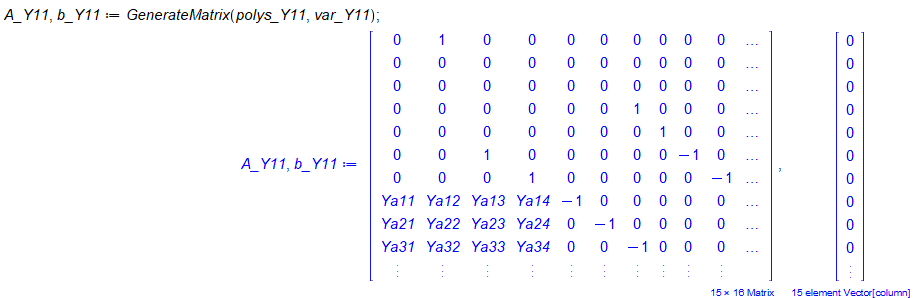and solve it with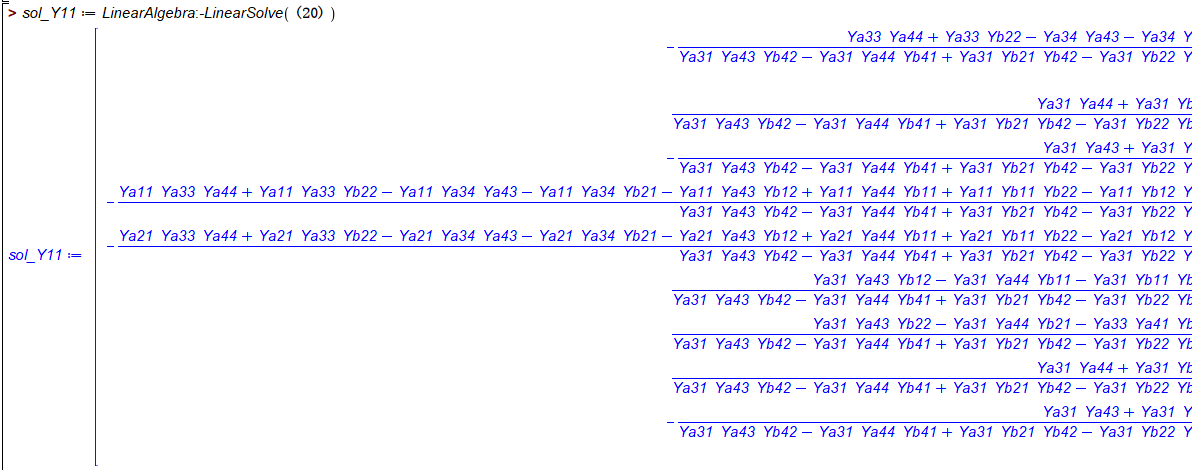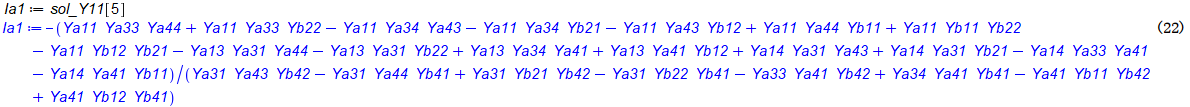what I expected is Ia1=f(Va1) which Va1 as indepentent,but the above solution have no Va1
Question2: is the (22) give the right answer for Ia1 ? what is the indepent var for the above solution?

here is my full maple worksheet

CoupleLineSeriesConnect_Question.mw

best regards

## How can I distinguish between the components of a ...

Good Day.

I have attached a worksheet for a time series that comprises 45 data points. I would like to investigate the overall demand (for forecasting purposes) and to isolate the underlying demand components; that is, trend, level, seasonal, and residual. However, I have difficulty in distinguishing each component in the plot as the colors appear to be similar and some may have relatively small values.

Does anyone know how to recolor these components so they appear to be more obvious? It would be also beneficial to isolate and plot each individual component - can that be done?

MaplePrimes_Time_Series.mw

## Hypergraphs Package any...

Anyone know about a Hypergraph package for maple like

HyperNetX in Python

https://pnnl.github.io/HyperNetX/build/overview/index.html

Is their anyway we can use python packages in maple

## How to install Maple in my new laptop...

Hello:

I've changed my laptop this week and I'd like to know how to install Maple with my account in the new one.

Sergio Sanz.

## complex functions...

hi everybody, i have a question, i hope u can help me, i want to know how to convert a complex function to conjugate form, for example i want to convert  exp(Ix)  to exp(-Ix) when x is real. conjugate  command dosn't work for me, becuse it assume  that we dont khow x is real or not,when i use assume command befor conjugate,again it does not  solve my problem, in the other hand, evalc command dosn't work too, becuse i want to have exponential form. thanks so much

## Deteriorated performance in Maple's new versions ...

I have some maple procedures that worked fine for many years. However, I started to notice some important performance deterioration with the new versions (Maple 2021 and 2022). I could identify the "culprit", they are some simplify/sum commands like this one:

```A := simplify( sum( sum( sum( sum(sum( sum( sum( sum( ((x-i1)^2+(y-i2)^2)*((x-j1)^2+(y-j2)^2)*((x-k1)^2+(y-k2)^2)*((x-l1)^2+(y-l2)^2) ,i1=1..N) ,j1=1..N),k1=1..N),l1=1..N) ,i2=1..N),j2=1..N),k2=1..N),l2=1..N) );```

Using, for instance, Maple 2017, this command requires to complete:

N:=4
memory used=258.2MB, alloc=44.3MB, time=1.58
N:=6
memory used=5247.1MB, alloc=394.0MB, time=39.35
N:=7
memory used=16405.4MB, alloc=775.9MB, time=135.78

On the other hand, in Maple 2022 (similar results for 2021), we have

N:=4
memory used=527.7MB, alloc=44.3MB, time=3.85
N := 6
memory used=10763.4MB, alloc=409.6MB, time=97.22
N := 7
memory used=34564.2MB, alloc=1139.9MB, time=351.94

My laptop (16G RAM) cannot execute N:=8 in Maple 2022 in reasonable time, I guess due to RAM limitation.

Does someone have any hint on how to improve the performance of these commands in Maple newer versions?

﻿